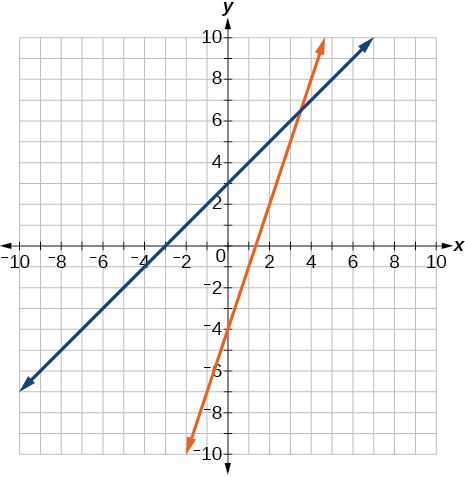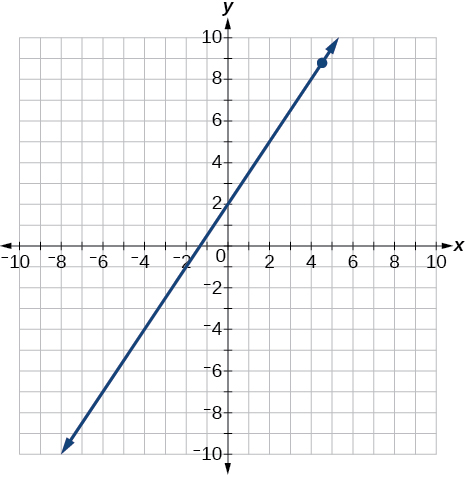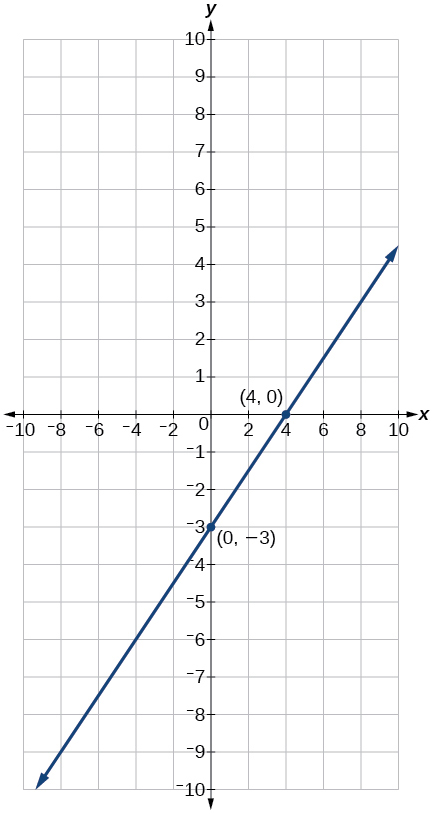2.7 Linear inequalities and absolute value inequalities  (Page 7/11)

 Page 7 / 11

A man has 72 ft. of fencing to put around a rectangular garden. If the length is 3 times the width, find the dimensions of his garden.

A truck rental is $25 plus$.30/mi. Find out how many miles Ken traveled if his bill was $50.20. 84 mi Complex Numbers For the following exercises, use the quadratic equation to solve. ${x}^{2}-5x+9=0$ $2{x}^{2}+3x+7=0$ $x=\frac{-3}{4}±\frac{i\sqrt{47}}{4}$ For the following exercises, name the horizontal component and the vertical component. $4-3i$ $-2-i$ horizontal component $\text{\hspace{0.17em}}-2;$ vertical component $\text{\hspace{0.17em}}-1$ For the following exercises, perform the operations indicated. $\left(9-i\right)-\left(4-7i\right)$ $\left(2+3i\right)-\left(-5-8i\right)$ $7+11i$ $2\sqrt{-75}+3\sqrt{25}$ $\sqrt{-16}+4\sqrt{-9}$ $16i$ $-6i\left(i-5\right)$ ${\left(3-5i\right)}^{2}$ $-16-30i$ $\sqrt{-4}·\sqrt{-12}$ $\sqrt{-2}\left(\sqrt{-8}-\sqrt{5}\right)$ $-4-i\sqrt{10}$ $\frac{2}{5-3i}$ $\frac{3+7i}{i}$ $x=7-3i$ Quadratic Equations For the following exercises, solve the quadratic equation by factoring. $2{x}^{2}-7x-4=0$ $3{x}^{2}+18x+15=0$ $x=-1,-5$ $x=0,\frac{9}{7}$ For the following exercises, solve the quadratic equation by using the square-root property. ${x}^{2}=49$ ${\left(x-4\right)}^{2}=36$ $x=10,-2$ For the following exercises, solve the quadratic equation by completing the square. ${x}^{2}+8x-5=0$ $4{x}^{2}+2x-1=0$ $x=\frac{-1±\sqrt{5}}{4}$ For the following exercises, solve the quadratic equation by using the quadratic formula. If the solutions are not real, state No real solution . $2{x}^{2}-5x+1=0$ $15{x}^{2}-x-2=0$ $x=\frac{2}{5},\frac{-1}{3}$ For the following exercises, solve the quadratic equation by the method of your choice. ${\left(x-2\right)}^{2}=16$ ${x}^{2}=10x+3$ $x=5±2\sqrt{7}$ Other Types of Equations For the following exercises, solve the equations. ${x}^{\frac{3}{2}}=27$ ${x}^{\frac{1}{2}}-4{x}^{\frac{1}{4}}=0$ $x=0,256$ $4{x}^{3}+8{x}^{2}-9x-18=0$ $3{x}^{5}-6{x}^{3}=0$ $x=0,±\sqrt{2}$ $\sqrt{x+9}=x-3$ $\sqrt{3x+7}+\sqrt{x+2}=1$ $x=-2$ $|3x-7|=5$ $|2x+3|-5=9$ $x=\frac{11}{2},\frac{-17}{2}$ Linear Inequalities and Absolute Value Inequalities For the following exercises, solve the inequality. Write your final answer in interval notation. $5x-8\le 12$ $-2x+5>x-7$ $\left(-\infty ,4\right)$ $\frac{x-1}{3}+\frac{x+2}{5}\le \frac{3}{5}$ $|3x+2|+1\le 9$ $\left[\frac{-10}{3},2\right]$ $|5x-1|>14$ $|x-3|<-4$ No solution For the following exercises, solve the compound inequality. Write your answer in interval notation. $-4<3x+2\le 18$ $3y<1-2y<5+y$ $\left(-\frac{4}{3},\frac{1}{5}\right)$ For the following exercises, graph as described. Graph the absolute value function and graph the constant function. Observe the points of intersection and shade the x -axis representing the solution set to the inequality. Show your graph and write your final answer in interval notation. $|x+3|\ge 5$ Graph both straight lines (left-hand side being y1 and right-hand side being y2) on the same axes. Find the point of intersection and solve the inequality by observing where it is true comparing the y -values of the lines. See the interval where the inequality is true. $x+3<3x-4$ Where the blue is below the orange line; point of intersection is $\text{\hspace{0.17em}}x=3.5.$ $\left(3.5,\infty \right)$Chapter practice test Graph the following: $\text{\hspace{0.17em}}2y=3x+4.$ $y=\frac{3}{2}x+2$ x y 0 2 2 5 4 8Find the x- and y -intercepts for the following: $2x-5y=6$ Find the x- and y -intercepts of this equation, and sketch the graph of the line using just the intercepts plotted. $3x-4y=12$ $\left(0,-3\right)$ $\left(4,0\right)$Find the exact distance between $\text{\hspace{0.17em}}\left(5,-3\right)\text{\hspace{0.17em}}$ and $\text{\hspace{0.17em}}\left(-2,8\right).\text{\hspace{0.17em}}$ Find the coordinates of the midpoint of the line segment joining the two points. Write the interval notation for the set of numbers represented by $\text{\hspace{0.17em}}\left\{x|x\le 9\right\}.$ $\left(-\infty ,9\right]$ Solve for x : $\text{\hspace{0.17em}}5x+8=3x-10.$ Solve for x : $\text{\hspace{0.17em}}3\left(2x-5\right)-3\left(x-7\right)=2x-9.$ $x=-15$ Solve for x : $\text{\hspace{0.17em}}\frac{x}{2}+1=\frac{4}{x}$ Solve for x : $\text{\hspace{0.17em}}\frac{5}{x+4}=4+\frac{3}{x-2}.$ $x\ne -4,2;$ $x=\frac{-5}{2},1$ The perimeter of a triangle is 30 in. The longest side is 2 less than 3 times the shortest side and the other side is 2 more than twice the shortest side. Find the length of each side. Solve for x . Write the answer in simplest radical form. $\frac{{x}^{2}}{3}-x=\frac{-1}{2}$ $x=\frac{3±\sqrt{3}}{2}$ Solve: $\text{\hspace{0.17em}}3x-8\le 4.$ Solve: $\text{\hspace{0.17em}}|2x+3|<5.$ $\left(-4,1\right)$ Solve: $\text{\hspace{0.17em}}|3x-2|\ge 4.$ For the following exercises, find the equation of the line with the given information. Passes through the points $\text{\hspace{0.17em}}\left(-4,2\right)\text{\hspace{0.17em}}$ and $\text{\hspace{0.17em}}\left(5,-3\right).$ $y=\frac{-5}{9}x-\frac{2}{9}$ Has an undefined slope and passes through the point $\text{\hspace{0.17em}}\left(4,3\right).$ Passes through the point $\text{\hspace{0.17em}}\left(2,1\right)\text{\hspace{0.17em}}$ and is perpendicular to $\text{\hspace{0.17em}}y=\frac{-2}{5}x+3.$ $y=\frac{5}{2}x-4$ Add these complex numbers: $\text{\hspace{0.17em}}\left(3-2i\right)+\left(4-i\right).$ Simplify: $\text{\hspace{0.17em}}\sqrt{-4}+3\sqrt{-16}.$ $14i$ Multiply: $\text{\hspace{0.17em}}5i\left(5-3i\right).$ Divide: $\text{\hspace{0.17em}}\frac{4-i}{2+3i}.$ $\frac{5}{13}-\frac{14}{13}i$ Solve this quadratic equation and write the two complex roots in $\text{\hspace{0.17em}}a+bi\text{\hspace{0.17em}}$ form: $\text{\hspace{0.17em}}{x}^{2}-4x+7=0.$ Solve: $\text{\hspace{0.17em}}{\left(3x-1\right)}^{2}-1=24.$ $x=2,\frac{-4}{3}$ Solve: $\text{\hspace{0.17em}}{x}^{2}-6x=13.$ Solve: $\text{\hspace{0.17em}}4{x}^{2}-4x-1=0$ $x=\frac{1}{2}±\frac{\sqrt{2}}{2}$ Solve: $\sqrt{x-7}=x-7$ Solve: $\text{\hspace{0.17em}}2+\sqrt{12-2x}=x$ $4$ Solve: $\text{\hspace{0.17em}}{\left(x-1\right)}^{\frac{2}{3}}=9$ For the following exercises, find the real solutions of each equation by factoring. $2{x}^{3}-{x}^{2}-8x+4=0$ $x=\frac{1}{2},2,-2$ ${\left(x+5\right)}^{2}-3\left(x+5\right)-4=0$ Questions & Answers what is math number Tric Reply x-2y+3z=-3 2x-y+z=7 -x+3y-z=6 Sidiki Reply Need help solving this problem (2/7)^-2 Simone Reply x+2y-z=7 Sidiki what is the coefficient of -4× Mehri Reply -1 Shedrak the operation * is x * y =x + y/ 1+(x × y) show if the operation is commutative if x × y is not equal to -1 Alfred Reply An investment account was opened with an initial deposit of$9,600 and earns 7.4% interest, compounded continuously. How much will the account be worth after 15 years?
lim x to infinity e^1-e^-1/log(1+x)
given eccentricity and a point find the equiation
12, 17, 22.... 25th term
12, 17, 22.... 25th term
Akash
College algebra is really hard?
Absolutely, for me. My problems with math started in First grade...involving a nun Sister Anastasia, bad vision, talking & getting expelled from Catholic school. When it comes to math I just can't focus and all I can hear is our family silverware banging and clanging on the pink Formica table.
Carole
I'm 13 and I understand it great
AJ
I am 1 year old but I can do it! 1+1=2 proof very hard for me though.
Atone
hi
Not really they are just easy concepts which can be understood if you have great basics. I am 14 I understood them easily.
Vedant
find the 15th term of the geometric sequince whose first is 18 and last term of 387
I know this work
salma
The given of f(x=x-2. then what is the value of this f(3) 5f(x+1)
hmm well what is the answer
Abhi
If f(x) = x-2 then, f(3) when 5f(x+1) 5((3-2)+1) 5(1+1) 5(2) 10
Augustine
how do they get the third part x = (32)5/4
make 5/4 into a mixed number, make that a decimal, and then multiply 32 by the decimal 5/4 turns out to be
AJ
how
Sheref
can someone help me with some logarithmic and exponential equations.
20/(×-6^2)
Salomon
okay, so you have 6 raised to the power of 2. what is that part of your answer
I don't understand what the A with approx sign and the boxed x mean
it think it's written 20/(X-6)^2 so it's 20 divided by X-6 squared
Salomon
I'm not sure why it wrote it the other way
Salomon
I got X =-6
Salomon
ok. so take the square root of both sides, now you have plus or minus the square root of 20= x-6
oops. ignore that.
so you not have an equal sign anywhere in the original equation?
hmm
Abhi
is it a question of log
Abhi
🤔.
Abhi
I rally confuse this number And equations too I need exactly help
salma
But this is not salma it's Faiza live in lousvile Ky I garbage this so I am going collage with JCTC that the of the collage thank you my friends
salma
Commplementary angles
hello
Sherica
im all ears I need to learn
Sherica
right! what he said ⤴⤴⤴
Tamia
hii
Uday
hi
salma
hi
Ayuba
Hello
opoku
hi
Ali
greetings from Iran
Ali
salut. from Algeria
Bach
hi
NharnharByBy RhodesBy Prateek AshtikarByBy